﻿ 地铁列车智能吹扫系统研发

# 地铁列车智能吹扫系统研发The Research and Development of the Intelligent Purge System of the Subway Train

Abstract: The intelligent purge system of the subway train, including the intelligent purge and cleaning ro-bots on the underside and on both sides of the subway train, are equipped with magnetic naviga-tion sensors, and walk synchronously on the trench and the ground on both sides through laying the navigation magnetic strips; optical fiber locators are used to making sure the primal coordi-nate; the QR Code and the code reader is used to locate the key parts of the subway train, so as to realize the key cleaning of the parts with serious dust bunnies, and at the same time, the dust fall is synchronously collected, and can be discharged after being filtered to act the mustard, which will improve the working efficiency of purging, reduce labor intensity and improve the working environment of purging.

1. 背景介绍

2. 系统组成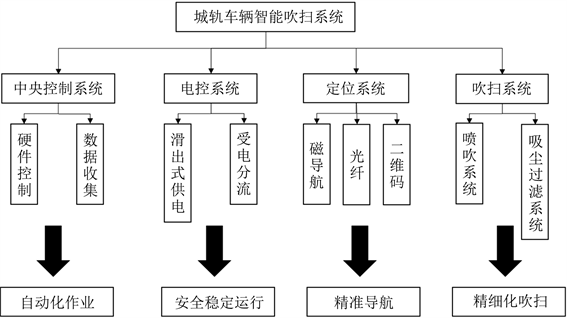Figure 1. The general structure of the intelligent purge system of the subway train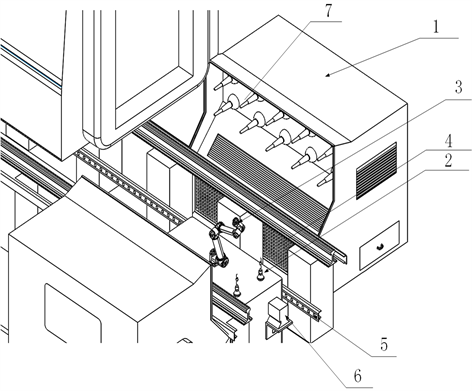Figure 2. The schematic diagram of the intelligent purge system of the subway train

3. 分部介绍

3.1. 中央控制系统

3.2. 电控系统

3.3. 定位系统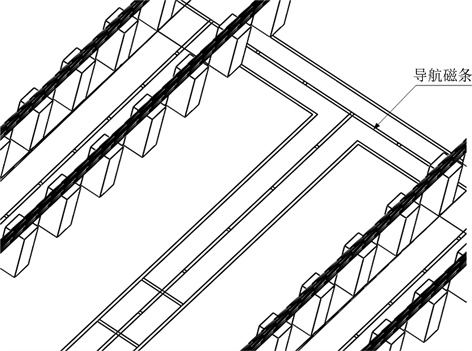Figure 3. The schematic diagram of the navigation magnetic strips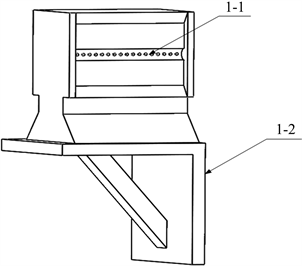Figure 4. The schematic diagram of the code reader

3.4. 吹扫系统

3.4.1. 吸尘口分析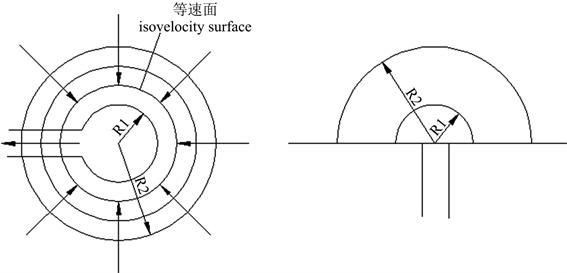(a)(b)

Figure 5. The schematic of point convergenceradial and equipotential surface of velocity

$Q=4\pi {r}_{1}^{2}{v}_{1}=4\pi {r}_{2}^{2}{v}_{2}$ (1)

v1、v2——球面1和2的气体流速，m/s；

r1、r2——球面1和2的半径，m。

$Q=2\pi {r}_{1}^{2}{v}_{1}=2\pi {r}_{2}^{2}{v}_{2}$ (2)

$\frac{{v}_{x}}{{v}_{0}}=\frac{1}{1+4.7{\left(\frac{{a}_{0}}{{b}_{0}}\right)}^{0.34}{\left(\frac{x}{\sqrt{{A}_{0}}}\right)}^{1.4}}$ (3)

vx——距离吸尘口x处的气体流动速度，m/s；

A0——吸尘口的截面面积，m2

x——吸口外部某点至吸尘口的间距，m；

a0、b0——矩形吸尘口长和宽，m；Table 1. The form of the minimum control wind velocity

$Q=3600×0.75×\left(5{x}^{2}+A\right){v}_{x}$ (4)

A——吸尘口面积，m2

vx——捕吸速度，m/s。

3.4.2. 喷嘴分析

${v}_{m}=\frac{2.4{v}_{0}}{\sqrt{\frac{\alpha s}{{b}_{0}}+0.41}}$ (5)

${Q}_{m}=\left(2.4\sqrt{\frac{\alpha s}{{b}_{0}}}+0.294\right){Q}_{0}$ (6)

α——射流紊流系数，扁射流α = 0.11~0.12；

s——断面距吹气口距离，mm；

b0——矩形吹气口宽度，mm；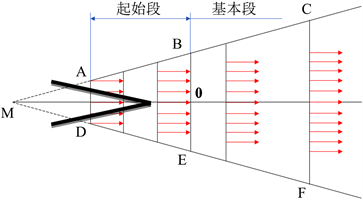Figure 6. The schematic of jet velocity distribution

${q}_{v}=18.298\alpha A\frac{\sqrt{{T}_{0}}{T}_{s}{p}_{0}}{{p}_{s}{T}_{0}}$ (7)

α——射流紊流系数，扁射流α = 0.11~0.12；

s——断面距吹气口距离，mm；

b0——矩形吹气口宽度，mm；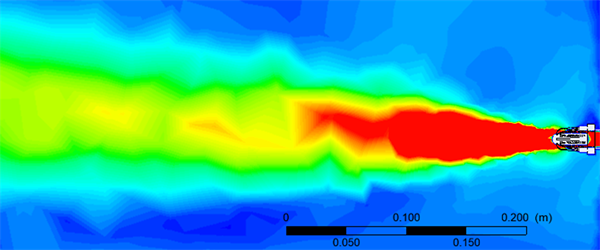Figure 7. The simulation charts of cluster nozzle air current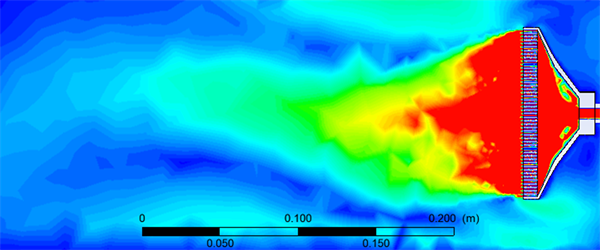Figure 8. The simulation charts of flat and horizontal nozzle air current

4. 实施方案

5. 结论

1) 通过三台城轨车辆智能吹扫机器人实现对车侧以及车底的吹扫，吹扫、吸尘过程功能同步进行，高压气流直接把所需清洁表面的粉尘、污垢吹起，吹起的尘粒被除尘器瞬间吸走过滤，避免了二次扬尘，降低了作业后扬尘的浓度。

2) 通过三台城轨车辆智能吹扫机器人实现同步过滤自清洁，进入除尘器的粉尘先进入除尘装置过滤，剩余尘粒进入高效过滤器过滤，净化后的空气达标排放，保护周围环境。

3) 通过在走行机构如AGV小车内装有磁导航传感器，可以实时感应铺设在地面的导航磁条发出的磁场信号，实现AGV小车在运行过程的精确定位；通过二维码和光纤定位的配合，可以由程序自动计算坐标，实现对车辆关键部位的重点定位，准确清扫；同时，码带对灰尘和损伤不敏感，更可靠、更实用、更耐用。

4) 通过三台城轨车辆智能吹扫机器人可实现在地沟内、外侧灵活移动，拥有柔性、灵活的机械手，改良除尘喷嘴，融合噪声屏蔽、吸音降噪技术，使工作环境更加人性化。

5) 通过三台城轨车辆智能吹扫机器人实现设备的控制一建智能化启动，依托大数据和算法程序的支持，在作业期间，无需人工参与，全程自动化完成，吹扫作业效率大大提高。

 刘海娇, 薛强, 卢晓东, 闫伯骏, 马麒, 朱兆立, 崔钰凡, 杜雅兰. 轨道车辆吹扫工艺现状分析[J]. 铁路节能环保与安全卫生, 2019, 9(6): 44-46+52.

 王贺. 地铁车辆除尘工艺设计方案研究[J]. 科学技术创新, 2020(17): 158-159.

 易健, 沃野. 地铁车辆密闭式吹扫除尘系统[J]. 电力机车与城轨车辆, 2017, 40(5): 77-79.

 杜雅兰, 姜立升, 马麒, 张帆. 粉尘在通风管道内沉降规律的研究[J]. 铁道劳动安全卫生与环保, 2005, 32(6): 275-279.

 何志平, 杜雅兰, 李广元, 王栋, 杨嘉鸿. 移动式排风净化装置在吹扫工艺的应用设计[J]. 铁路节能环保与安全卫生, 2017, 7(2): 101-103.

 刘旭东. 浅析地铁列车底部吹扫除尘系统[J]. 哈尔滨铁道科技, 2012(1): 3-4.

Top